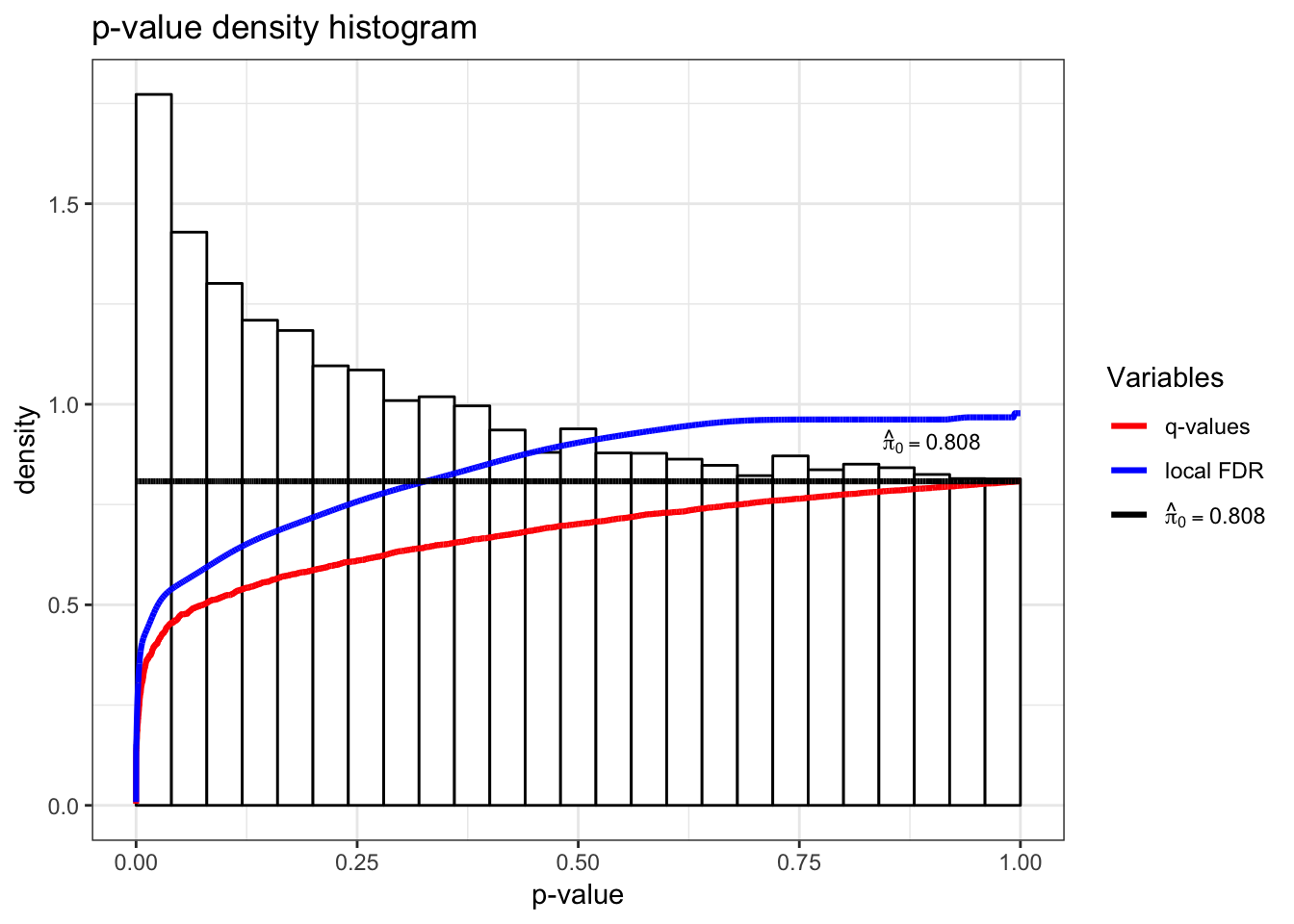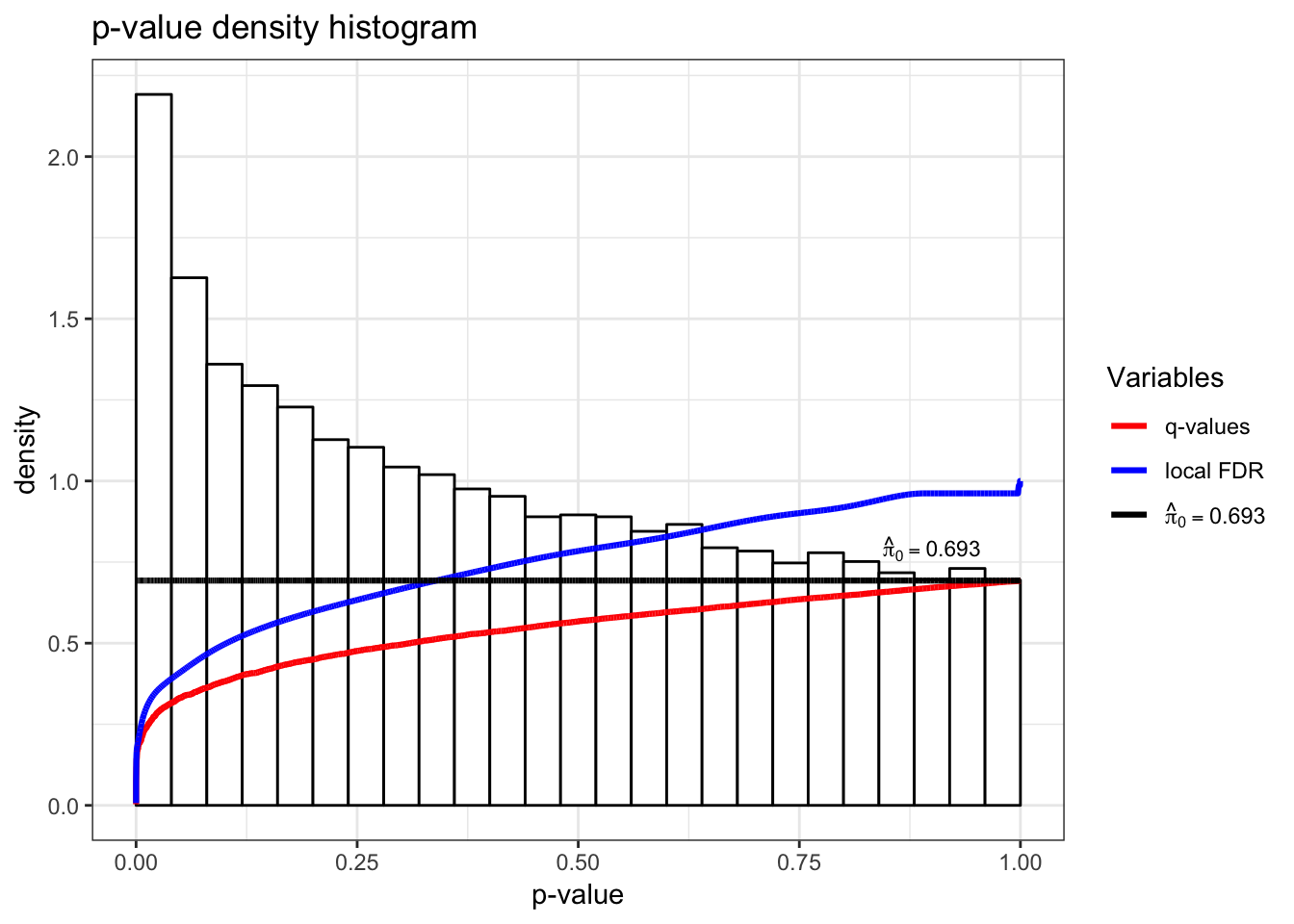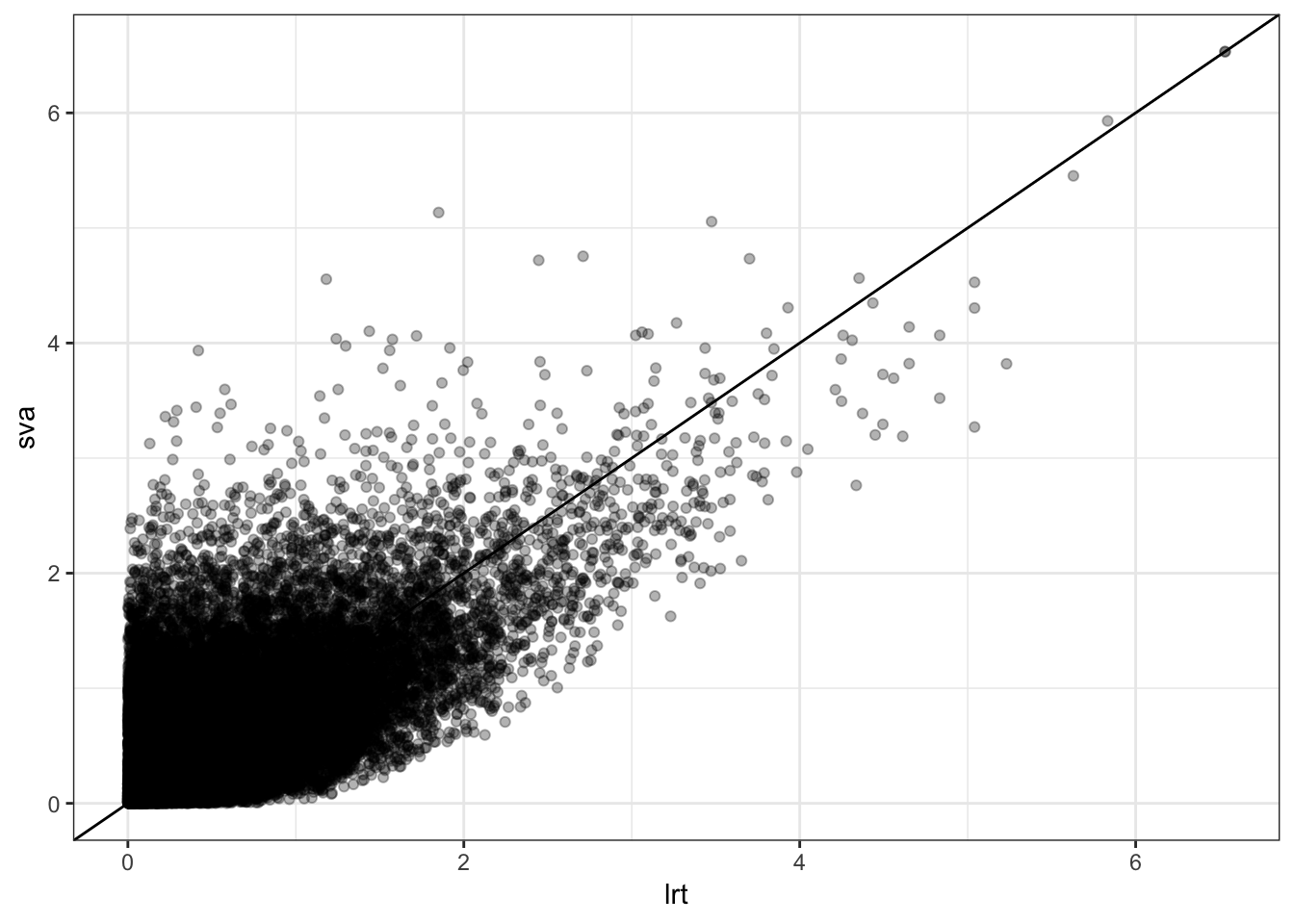# 79 Surrogate Variable Analysis

The surrogate variable analysis (SVA) model combines the many responses model with the latent variable model introduced above:

${\boldsymbol{Y}}_{m \times n} = {\boldsymbol{B}}_{m \times d} {\boldsymbol{X}}_{d \times n} + {\boldsymbol{\Phi}}_{m \times r} {\boldsymbol{Z}}_{r \times n} + {\boldsymbol{E}}_{m \times n}$

where $$m \gg n > d + r$$.

Here, only $${\boldsymbol{Y}}$$ and $${\boldsymbol{X}}$$ are observed, so we must combine many regressors model fitting techniques with latent variable estimation.

The variables $${\boldsymbol{Z}}$$ are called surrogate variables for what would be a complete model of all systematic variation.

## 79.1 Procedure

The main challenge is that the row spaces of $${\boldsymbol{X}}$$ and $${\boldsymbol{Z}}$$ may overlap. Even when $${\boldsymbol{X}}$$ is the result of a randomized experiment, there will be a high probability that the row spaces of $${\boldsymbol{X}}$$ and $${\boldsymbol{Z}}$$ have some overlap.

Therefore, one cannot simply estimate $${\boldsymbol{Z}}$$ by applying a latent variable esitmation method on the residuals $${\boldsymbol{Y}}- \hat{{\boldsymbol{B}}} {\boldsymbol{X}}$$ or on the observed response data $${\boldsymbol{Y}}$$. In the former case, we will only estimate $${\boldsymbol{Z}}$$ in the space orthogonal to $$\hat{{\boldsymbol{B}}} {\boldsymbol{X}}$$. In the latter case, the estimate of $${\boldsymbol{Z}}$$ may modify the signal we can estimate in $${\boldsymbol{B}}{\boldsymbol{X}}$$.

A recent method, takes an EM approach to esitmating $${\boldsymbol{Z}}$$ in the model

${\boldsymbol{Y}}_{m \times n} = {\boldsymbol{B}}_{m \times d} {\boldsymbol{X}}_{d \times n} + {\boldsymbol{\Phi}}_{m \times r} {\boldsymbol{Z}}_{r \times n} + {\boldsymbol{E}}_{m \times n}.$

It is shown to be necessary to penalize the likelihood in the estimation of $${\boldsymbol{B}}$$ — i.e., form shrinkage estimates of $${\boldsymbol{B}}$$ — in order to properly balance the row spaces of $${\boldsymbol{X}}$$ and $${\boldsymbol{Z}}$$.

The regularized EM algorithm, called cross-dimensonal inference (CDI) iterates between

1. Estimate $${\boldsymbol{Z}}$$ from $${\boldsymbol{Y}}- \hat{{\boldsymbol{B}}}^{\text{Reg}} {\boldsymbol{X}}$$
2. Estimate $${\boldsymbol{B}}$$ from $${\boldsymbol{Y}}- \hat{{\boldsymbol{\Phi}}} \hat{{\boldsymbol{Z}}}$$

where $$\hat{{\boldsymbol{B}}}^{\text{Reg}}$$ is a regularized or shrunken estimate of $${\boldsymbol{B}}$$.

It can be shown that when the regularization can be represented by a prior distribution on $${\boldsymbol{B}}$$ then this algorithm achieves the MAP.

## 79.2 Example: Kidney Expr by Age

In Storey et al. (2005), we considered a study where kidney samples were obtained on individuals across a range of ages. The goal was to identify genes with expression associated with age.

> library(edge)
> library(splines)
> age <- kidcov$age > sex <- kidcov$sex
> dim(kidexpr)
 34061    72
> cov <- data.frame(sex = sex, age = age)
> null_model <- ~sex
> full_model <- ~sex + ns(age, df = 3)
> de_obj <- build_models(data = kidexpr, cov = cov,
+                        null.model = null_model,
+                        full.model = full_model)
> de_lrt <- lrt(de_obj, nullDistn = "bootstrap", bs.its = 100, verbose=FALSE)
> qobj1 <- qvalueObj(de_lrt)
> hist(qobj1)Now that we have completed a standard generalized LRT, let’s estimate $${\boldsymbol{Z}}$$ (the surrogate variables) using the sva package as accessed via the edge package.

> dim(nullMatrix(de_obj))
 72  2
> de_sva <- apply_sva(de_obj, n.sv=4, method="irw", B=10)
Number of significant surrogate variables is:  4
Iteration (out of 10 ):1  2  3  4  5  6  7  8  9  10
> dim(nullMatrix(de_sva))
 72  6
> de_svalrt <- lrt(de_sva, nullDistn = "bootstrap", bs.its = 100, verbose=FALSE)
> qobj2 <- qvalueObj(de_svalrt)
> hist(qobj2)> summary(qobj1)

Call:
qvalue(p = pval)

pi0:    0.8081212

Cumulative number of significant calls:

<1e-04 <0.001 <0.01 <0.025 <0.05 <0.1    <1
p-value       27    161   798   1676  2906 5271 34061
q-value        0      0     2      4    10   27 34061
local FDR      0      0     2      2     5   18 34061
> summary(qobj2)

Call:
qvalue(p = pval)

pi0:    0.6925105

Cumulative number of significant calls:

<1e-04 <0.001 <0.01 <0.025 <0.05 <0.1    <1
p-value       28    151  1001   2051  3549 6168 34061
q-value        0      0     3      4     6   51 34061
local FDR      0      0     2      2     3   28 34053

P-values from two analyses are fairly different.

> data.frame(lrt=-log10(qobj1$pval), sva=-log10(qobj2$pval)) %>%
+   ggplot() + geom_point(aes(x=lrt, y=sva), alpha=0.3) + geom_abline()